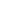#Mohan invested an amount of Rs. 7500 divided in two different schemes P and Q at the simple interest rate of 8% p.a. and 12% p.a. respectively. If the total amount of simple interest earned in 3 years be Rs. 2400, what was the amount invested in Scheme Q?
a) 5000
b) 2500
c) 3230
d) 2777

 5000. Solution Let the Sum invested in Scheme P be Rs. x and that in Scheme Q be Rs. (7500 - x). Then,(x x 8 x 3)/100 + (7500 - x) x 12 x 3/100 = 2400 ==> x = 2500. So, sum invested in Scheme Q = Rs. (7500 - 2500) = Rs. 5000
Share :
+
Next
« Prev
Prev
Next »